Arithmetic
Associative Property
Averages
Brackets
Closure Property
Commutative Property
Conversion of Measurement Units
Cube Root
Decimal
Divisibility Principles
Equality
Exponents
Factors
Fractions
Fundamental Operations
H.C.F / G.C.D
Integers
L.C.M
Multiples
Multiplicative Identity
Multiplicative Inverse
Numbers
Percentages
Profit and Loss
Ratio and Proportion
Simple Interest
Square Root
Unitary Method
Algebra
Cartesian System
Order Relation
Polynomials
Probability
Standard Identities & their applications
Transpose
Geometry
Basic Geometrical Terms
Circle
Curves
Angles
Define Line, Line Segment and Rays
Non-Collinear Points
Parallelogram
Rectangle
Rhombus
Square
Three dimensional object
Trapezium
Triangle
Trigonometry
Trigonometry Ratios
Data-Handling
Arithmetic Mean
Frequency Distribution Table
Graphs
Median
Mode
Range

Solved Problems
Home >> Triangle >> Properties >> Triangles on same base & between same parallel lines >>

## Triangles on the same base and between same parallel lines

 Sum of Two Sides Angle Sum Property Angles opposite to equal sides of triangle are equal Angle opposite to longer side is greater Pythagoras Theorem Exterior Angle Property of a Triangle Mid point property of Triangle Triangles on same base & between same parallel lines

 Before you understand this topic, you are advised to read: What is Parallelogram ? What are Parallel Lines ? What are Congruent figures ? What are the rules of Congruent Triangle ? What are Transversal Lines ? What are Corresponding Angles ? What is Angle Sum Property of Triangle ? This is one of the properties of triangle which says Triangles on the same base and between same parallel lines are equal in area Let's first understand how this situation looks like Observe the following diagram having two triangles - △ ABC and △ BCD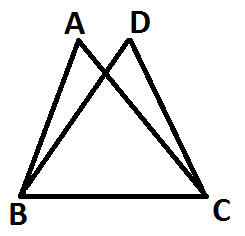Observe carefully and you can notice that in the above diagram there are two triangles (as shown below):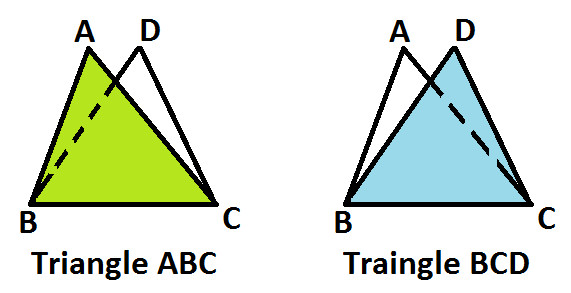Also, you can notice that both the triangle ABC and BCD are on the same base BC (as shown below)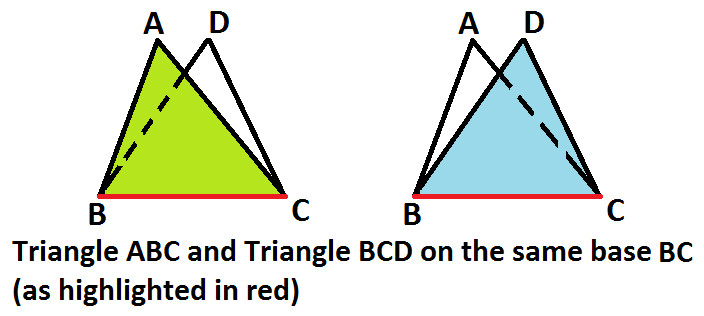Now, if you join vertices A & D with a line XY, you will notice that BC is parallel to XY. And both the triangles ABC and BCD are between same parallel lines BC and XY (as shown below)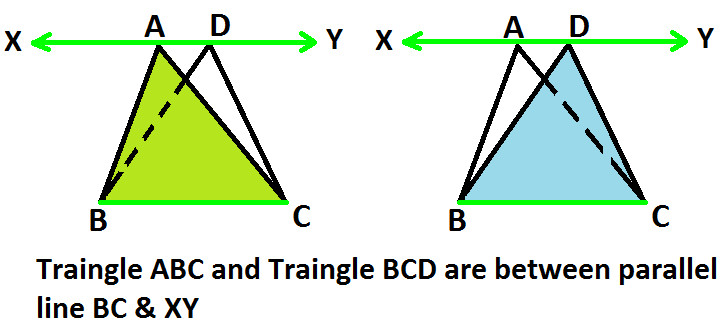Hence, you can see how triangles can be on same base and between same parallel lines. Now, let's prove this property that areas of triangles on the same base and between same parallel lines are same. i.e. Area of △ ABC = Area of △ BCD Observe the following diagram:Join vertices A & D with a line XY, you will notice that BC is parallel to XY (as shown below):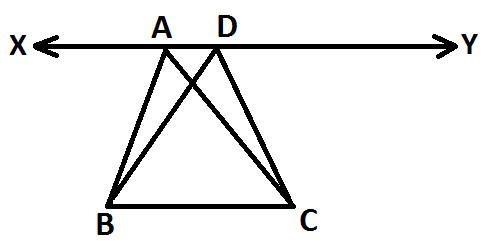From Vertex C, draw a line parallel to AB which meets line XY at point E and we get: AB is parallel to CE (as shown below)From Vertex C, draw another line parallel to BD which meets line XY at point F and we get: BD is parallel to CF (as shown below)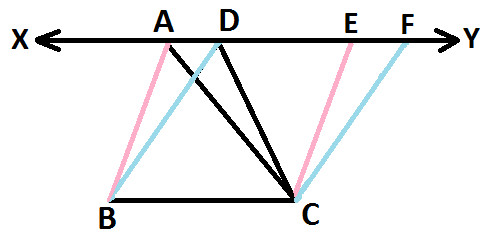Since XY is parallel to BC and AB is parallel to CE, so we get: Parallelogram ABCE (as shown below):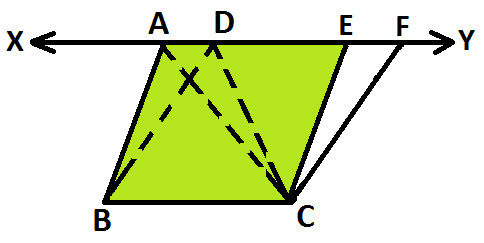In parallelogram ABCE, AC is a diagonal (as highlighted in red in below diagram):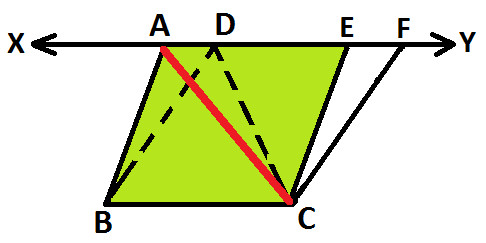Now, as we know that: Diagonals of a Parallelogram, divides it into two congruent triangles ( Diagonals of a parallelogram divide it into two congruent triangles ) So, we have △ ABC ≅ △ ACE (as shown below):Since, we know that congruent figures are equal in area, so we get: Area of △ ABC = Area of △ ACE ..... (statement 1) Now we know that: Area of Parallelogram ABCE = Area of △ ABC + Area of △ ACE From Statement 1: Area of △ ABC = Area of △ ACE, so we get: Area of Parallelogram ABCE = Area of △ ABC + Area of △ ABC Solve L.H.S and we get: Area of Parallelogram ABCE = 2 Area of △ ABC ..... (statement 2) Similarly, since XY is parallel to BC and BD is parallel to CF, so we get: Parallelogram BDFC (as shown below):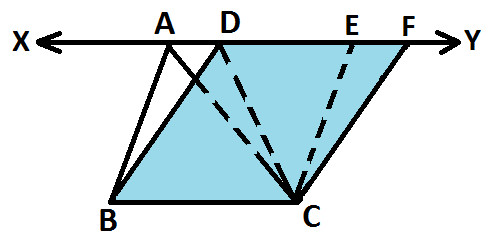In parallelogram BDFC, DC is a diagonal (as highlighted in red in below diagram):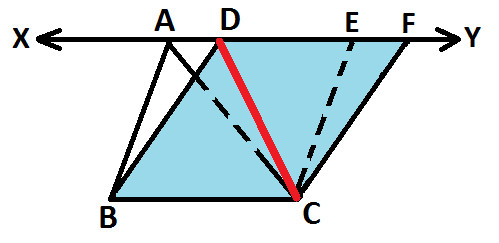Now, as we know that: Diagonals of a Parallelogram, divides it into two congruent triangles ( Diagonals of a parallelogram divide it into two congruent triangles ) So, we have △ BCD ≅ △ DCF (as shown below):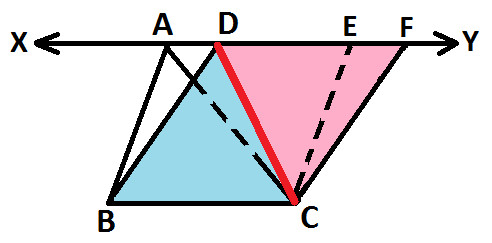Since, we know that congruent figures are equal in area, so we get: Area of △ BCD = Area of △ DCF ..... (statement 3) Now we know that: Area of Parallelogram BDFC = Area of △ BCD + Area of △ DCF From Statement 1: Area of △ BCD = Area of △ DCF, so we get: Area of Parallelogram ABCE = Area of △ BCD + Area of △ BCD Solve L.H.S and we get: Area of Parallelogram ABCE = 2 Area of △ BCD ..... (statement 4) Now, you can also observe that Parallelogram ABCE and Parallelogram BDFC are between same base BC and between same parallel lines BC and XY (as shown below):Here we applied the property of Parallelogram that: Parallelograms on the same base between same parallel lines are equal in areas and we get: ( Parallelogram on the same base and between same parallel lines ) Area of Parallelogram ABCE = Area of Parallelogram BDCF In statement 2 and 4 above we proved that: Area of Parallelogram ABCE = 2 Area of △ ABC Area of Parallelogram BDFC = 2 Area of △ BCD So replacing these values we get: 2 Area of △ ABC = 2 Area of △ BCD Dividing both side by 2 and we get: Area of △ ABC = Area of △ BCD (as shown below):This is how this property of triangle is obtained. From this property, we get the converse of this property which also stand true: Two Triangles on the same base and equal in areas lie between the same parallel lines